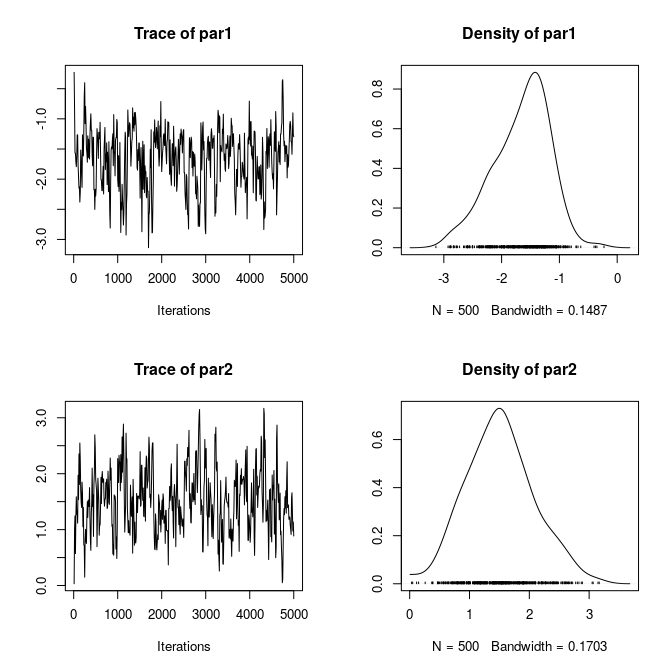# Extending ergmito

#### 2020-02-12

The ergmito’s workhorse are two other functions: (1) ergm’s ergm.allstats which is used to compute the support of model’s sufficient statistics, and (2) ergmito_formulae which is a wrapper of that same function, and that returns a list including the following two functions: loglike and grad, the functions to calculate the joint log-likelihood of the model and its gradient.

library(ergmito)
data(fivenets)
model_object <- ergmito_formulae(fivenets ~ edges + ttriad)

# Printing the model object
model_object
#> ergmito log-likelihood function
#> Number of networks:  5
#> Model:  fivenets ~ edges + ttriad
#> Available elements by using the $operator: #> loglik: function (params, ...) grad : function (params, ...) # Printing the log-likelihood function model_object$loglik
#> function (params, ...)
#> {
#>     ans <- sum(exact_loglik(ergmito_ptr, params = params, ...))
#>     if (!is.finite(ans))
#>         return(-.Machine$double.xmax * 1e-100) #> else return(ans) #> } #> <bytecode: 0x55a036f52f38> #> <environment: 0x55a036f54a90> Besides of the log-likelihood function and the gradient function, ergmito_formulae also returns We can take a look at each component from our previous object: # The vectors of weights str(model_object$stats.weights)
#> List of 5
#>  $: int [1:40] 66 12 168 72 48 196 120 1 88 1 ... #>$ : int [1:40] 66 12 168 72 48 196 120 1 88 1 ...
#>  $: int [1:40] 66 12 168 72 48 196 120 1 88 1 ... #>$ : int [1:40] 66 12 168 72 48 196 120 1 88 1 ...
#>  $: int [1:40] 66 12 168 72 48 196 120 1 88 1 ... # The matrices of the sufficient statistics str(model_object$stats.statmat)
#> List of 5
#>  $: num [1:40, 1:2] 2 1 4 8 7 3 6 12 6 0 ... #> ..- attr(*, "dimnames")=List of 2 #> .. ..$ : NULL
#>   .. ..$: chr [1:2] "edges" "ttriple" #>$ : num [1:40, 1:2] 2 1 4 8 7 3 6 12 6 0 ...
#>   ..- attr(*, "dimnames")=List of 2
#>   .. ..$: NULL #> .. ..$ : chr [1:2] "edges" "ttriple"
#>  $: num [1:40, 1:2] 2 1 4 8 7 3 6 12 6 0 ... #> ..- attr(*, "dimnames")=List of 2 #> .. ..$ : NULL
#>   .. ..$: chr [1:2] "edges" "ttriple" #>$ : num [1:40, 1:2] 2 1 4 8 7 3 6 12 6 0 ...
#>   ..- attr(*, "dimnames")=List of 2
#>   .. ..$: NULL #> .. ..$ : chr [1:2] "edges" "ttriple"
#>  $: num [1:40, 1:2] 2 1 4 8 7 3 6 12 6 0 ... #> ..- attr(*, "dimnames")=List of 2 #> .. ..$ : NULL
#>   .. ..$: chr [1:2] "edges" "ttriple" # The target statistic model_object$target.stats
#>      edges ttriple
#> [1,]     2       0
#> [2,]     7       4
#> [3,]     4       0
#> [4,]     5       2
#> [5,]     2       0

All this is closely related to the output object from the function ergm.allstats. The next section shows how all this works together to estimate the model parameters using Metropolis-Hastings MCMC.

# Example: Bayesian inference with fivenets

Suppose that we have a prior regarding the distribution of the fivenets model: The edges parameter is normally distributed with mean -1 and variance 2, while the nodematch("female") term has the same distribution but with mean 1. We can implement this model using a Metropolis-Hastings ratio. First, we need to define the log of the posterior distribution:

# Analyzing the model
model_object <- ergmito_formulae(fivenets ~ edges + nodematch("female"))
#> Warning: set_attrs() is deprecated as of rlang 0.3.0
#> This warning is displayed once per session.

# Defining the logposterior
logposterior <- function(p) {
model_object\$loglik(params = p) +
sum(dnorm(p, mean = c(-1,1), sd = sqrt(2), log = TRUE))
}


For this example, we are using the fmcmc R package. Here is how we put everything together:

# Loading the required R packages
library(fmcmc)
library(coda)

# Is it working?
logposterior(c(-1, 1))
#>  -38.24283

# Now, calling the MCMC function from the fmcmc R package
ans <- MCMC(
fun     = logposterior,
initial = c(0, 0),
# 5,000 steps sampling one of every ten iterations
nsteps  = 5000,
thin    = 10,
# We are using a normal transition kernel with .5 scale and updates are done
# one variable at a time in a random order
kernel = kernel_normal(scale = .5, scheme = "random")
)

We can now visualize our results. Since the resulting object is of class mcmc.list, which is implemented in the coda R package for MCMC diagnostics, we can use all the methods included in the package:

plot(ans)summary(ans)
#>
#> Iterations = 10:5000
#> Thinning interval = 10
#> Number of chains = 1
#> Sample size per chain = 500
#>
#> 1. Empirical mean and standard deviation for each variable,
#>    plus standard error of the mean:
#>
#>        Mean     SD Naive SE Time-series SE
#> par1 -1.655 0.4861  0.02174        0.04869
#> par2  1.514 0.5627  0.02517        0.05434
#>
#> 2. Quantiles for each variable:
#>
#>         2.5%    25%    50%    75%   97.5%
#> par1 -2.7733 -1.969 -1.574 -1.308 -0.8676
#> par2  0.5088  1.133  1.501  1.879  2.6639

Finally, we can compare this result with what we obtain from the ergmito function

summary(ergmito(fivenets ~ edges + nodematch("female")))
#>
#> ERGMito estimates
#>
#> formula:  fivenets ~ edges + nodematch("female")
#>
#>                  Estimate Std. Error z value Pr(>|z|)
#> edges            -1.70475    0.54356 -3.1363 0.001711 **
#> nodematch.female  1.58697    0.64305  2.4679 0.013592 *
#> ---
#> Signif. codes:  0 '***' 0.001 '**' 0.01 '*' 0.05 '.' 0.1 ' ' 1
#> AIC: 73.34109    BIC: 77.52978    (Smaller is better.)